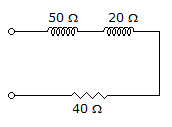# Electronics - RL Circuits - Discussion

### Discussion :: RL Circuits - True or False (Q.No.2)

2.The impedance of the circuit in the given circuit is 110.

 [A]. True [B]. False

Answer: Option B

Explanation:

No answer description available for this question.

 Vipul said: (Mar 6, 2014) z = sqrt[(xl1+xl2)^2+r^2]. = sqrt[(50+20)^2 + 40^2. = sqrt[4900+1600]. = 80.62 ohm.

 Simran said: (Jun 27, 2015) Why there is a square of resistance?

 Asma Asghar Janjua said: (Jun 18, 2016) I think so, in this case, the answer is false. The one end of the resistor becomes is floating so it is not included in finding impedance r = 0. Z = sqrt[(50+20) ^2]. Z = 70 ohm.

 Rao said: (Jan 4, 2021) It should be Z= √[R^2 + (Xl-XC) ^2].

#### Post your comments here:

Name *:

Email   : (optional)

» Your comments will be displayed only after manual approval.

#### Current Affairs 2021

Interview Questions and Answers